Question-and-Answer Resource for the Building Energy Modeling Community
Get s tarted with the Help page

# Infiltration in accordance with ASHRAE 90.1-2013, 2016 and 2019

I saw this very useful post and also read "Infiltration Modeling Guidelines for Commercial Building Energy Analysis", but I'm still not sure how everyone models Infiltration in accordance with ASHRAE 90.1 Appendix G.

I will introduce my approach below, so could you please give me your opinion on whether it is appropriate or not?

Beginning with the 2013 edition, ASHRAE 90.1 Appendix G specifies Infiltration (Air leakage) by the following formula: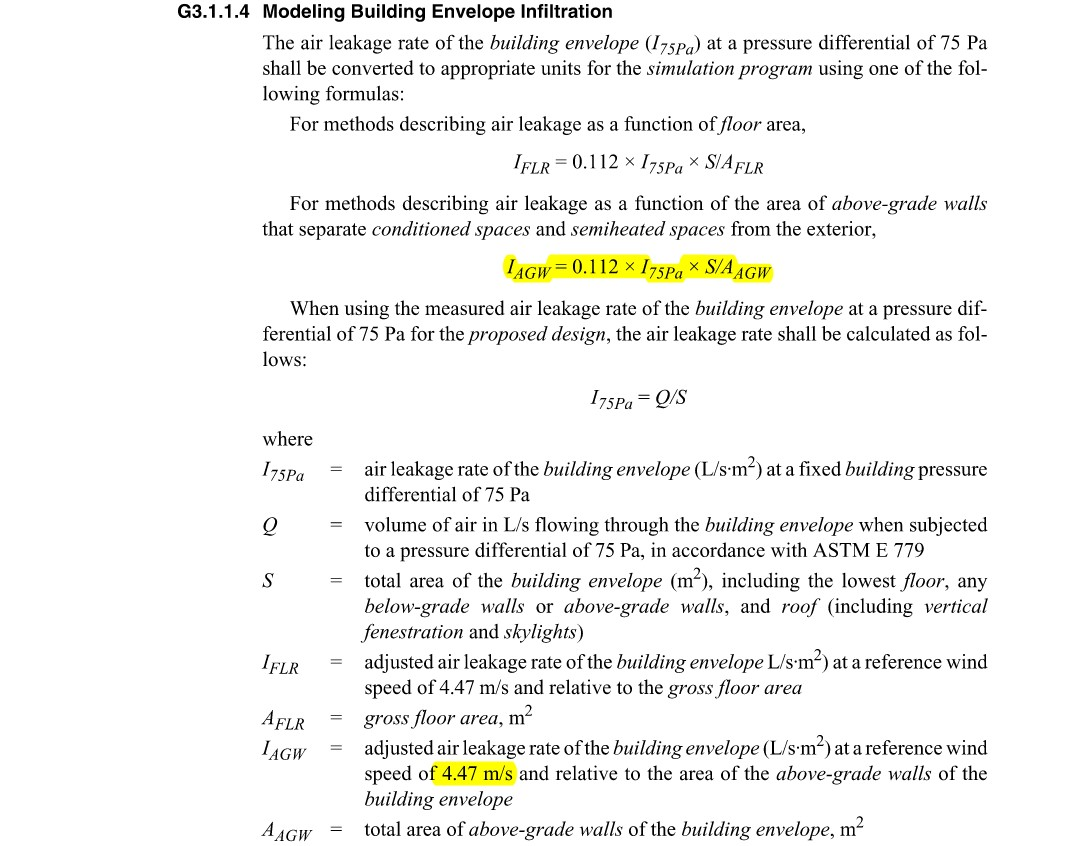S and AAGW are different for each project, and I75Pa varies slightly depending on the edition of 90.1 (In the 2013 and 2016 edition, it's 2.03 L/s·m2 for both baseline and proposed cases. In the 2019 edition, it's 5.1L/s·m2 for baseline case and 3.0L/s·m2 for proposed case). But anyway, IAGW is the adjusted infiltration at a reference wind speed of 4.47m/s, and the actual outdoor wind speed cannot always be 4.47m/s, so I think we need further adjustments to model Infiltration in EnergyPlus.

In EnergyPlus, ZoneInfiltration:DesignFlowRate uses the following equation.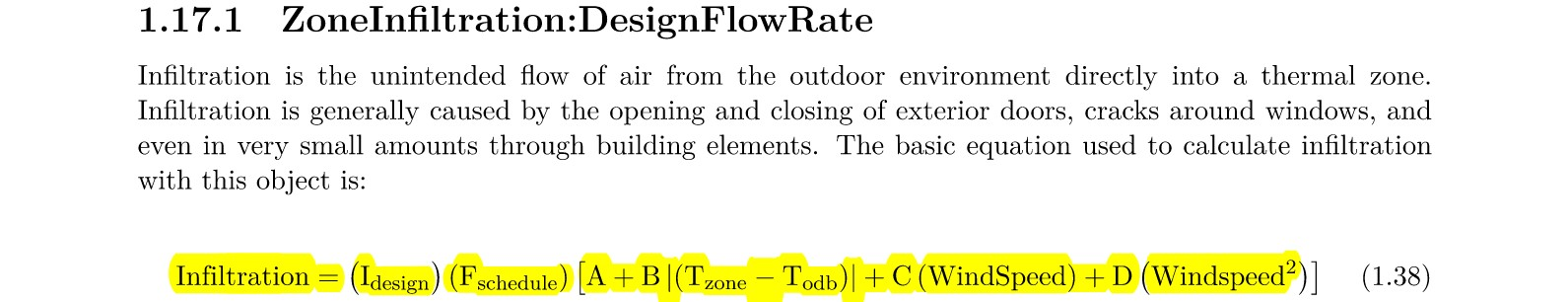Idesign can be IAGW. The four coefficients A, B, C and D needs to be input. I obtained them by regression analysis in Excel.

• For the WindSpeed, two cases were used for the regression analysis. One is at 4.47m/s and the Infiltration is equal to IAGW. The other is at 0m/s, in which case the Infiltration is assumed to be 0L/s·m2.
• For the Temperature difference between outdside and zones, I found "Table 802.1 Temperature Correction Factors for Pressurization and Depressurization Testing- Calculated according to ASTM E779-10" and I tried to utilise it at first, but as a result the regression analysis by Excel did not approximate Infiltration well. This is probably because the temperature difference term |Tzone - Todb| in the equation of Infiltration used in EnergyPlus is absolute value. I decided to ignore the temperature difference term, i.e., B=0.

I did regression analysis with just two data sets and got the following result.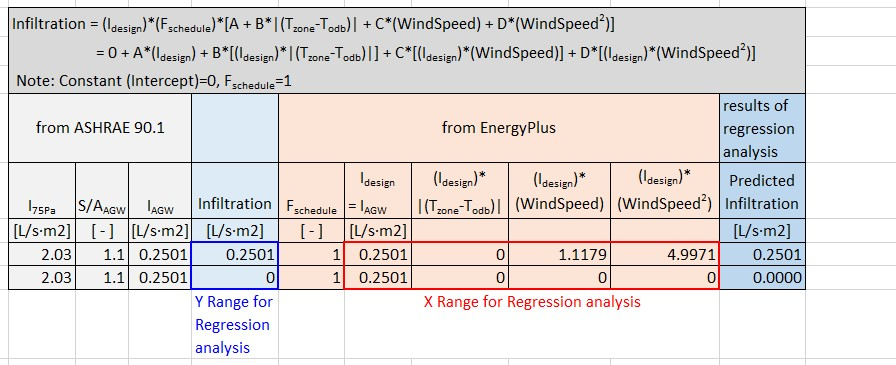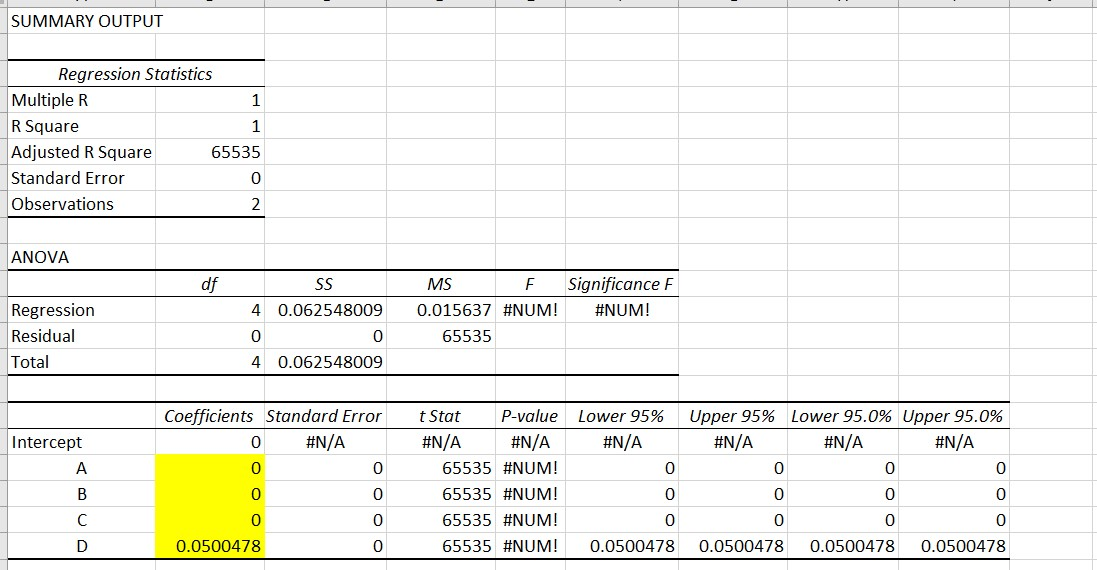A, B and C = 0, and the infiltration is proportional only to the square of the wind speed. Although this is different from any EnergyPlus, BLAST, or DOE-2 default setting, it seems to make some sense to me because dynamic pressure is proportional to the square of wind speed (P = 1/2* ρV^2). But I may be making some big mistakes or oversights. Any suggestions or advice on my procedure or results would be appreciated. I put my excel file here just in case.

edit retag close merge delete

Sort by » oldest newest most voted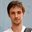If you want to model infiltration using Appendix G, I would suggest looking at the 90.1 Appendix G User Manual. The manual provides the coefficients. Essentially, since I_design is provided at 4.47 m/s, the adjustment factor should be 1 at these conditions. One way of getting there is to have C = 1/4.47 = 0.244 s/m (with A, B, D = 0). This is the value suggested in the User Manual.

I think your approach is fine (since 0.00500478 * 4.47^2 = 1) only if you assume that at non-design conditions (wind speed != 4.47 m/s) that the infiltration flow rate should be modified by the square of the wind speed.

more

1

Thank you for your quick reply. You are talking about not "Standard 90.1 User's Manual" but "ANSI/ASHRAE/IES Standard 90.1-2016 Performance Rating Method Reference Manual", right? Maybe I found the part what you mentioned. It says "The DOE-2 Infiltration methodology coefficients would be used, where: A = 0, B = 0, C = 0.224, D = 0" on page 3.30. Thank you so much!

Yes, that's it. Sorry, I should have provided a link.